# GSEB Solutions Class 8 Maths Chapter 1 Rational Numbers Ex 1.1

Gujarat Board GSEB Textbook Solutions Class 8 Maths Chapter 1 Rational Numbers Textbook Questions and Answers.

## Gujarat Board Textbook Solutions Class 8 Maths Chapter 1 Rational Numbers Ex 1.1

Question 1.
Using appropriate properties find:
(i) $$-\frac{2}{3} \times \frac{3}{5}+\frac{5}{2}-\frac{3}{5} \times \frac{1}{6}$$
(ii) $$\frac{2}{5} \times\left(-\frac{3}{7}\right)-\frac{1}{6} \times \frac{3}{2}+\frac{1}{14} \times \frac{2}{5}$$
Solution:
(i) $$-\frac{2}{3} \times \frac{3}{5}+\frac{5}{2}-\frac{3}{5} \times \frac{1}{6}$$
= $$\left[-\frac{2}{3} \times \frac{3}{5}+\left(\frac{-3}{5}\right) \times \frac{1}{6}\right]+\frac{5}{2}$$
(Using commutative)
= $$\left[-\frac{2}{3} \times \frac{3}{5}+\left(\frac{-3}{5}\right) \times \frac{1}{6}\right]+\frac{5}{2}$$ = $$\frac { 5 }{ 2 }$$
= $$\left(\frac{-3}{5}\right)\left[\frac{2}{3}+\frac{1}{6}\right]+\frac{5}{2}$$ (Using distributivity)
= $$\left(\frac{-3}{5}\right)\left[\frac{4+1}{6}\right]+\frac{5}{2}=\left(\frac{-3}{5}\right)\left[\frac{5}{6}\right]+\frac{5}{2}$$
= $$\frac{-3}{5} \times \frac{5}{6}+\frac{5}{2}=\frac{-1}{2}+\frac{5}{2}=\frac{-1+5}{2}=\frac{4}{2}$$ = 2

(ii) $$\frac{2}{5} \times\left(-\frac{3}{7}\right)-\frac{1}{6} \times \frac{3}{2}+\frac{1}{14} \times \frac{2}{5}$$
= $$\frac{2}{5} \times\left(\frac{-3}{7}\right)-\frac{1}{4}+\frac{1}{14} \times \frac{2}{5}$$
= $$\frac{2}{5} \times\left(\frac{-3}{7}\right)+\frac{1}{14} \times \frac{2}{5}-\frac{1}{4}$$
(Using commutative)
= $$\frac{2}{5}\left[\frac{-3}{7}+\frac{1}{14}\right]-\frac{1}{4}$$ (Using distributivity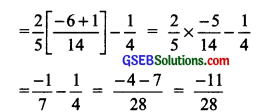Question 2.
Write the additive inverse of each of the following:

1. $$\frac { 2 }{ 8 }$$
2. $$\frac { -5 }{ 9 }$$
3. $$\frac { -6}{ -5 }$$
4. $$\frac { 2 }{ -9 }$$
5. $$\frac { 19 }{ -6 }$$

Solution: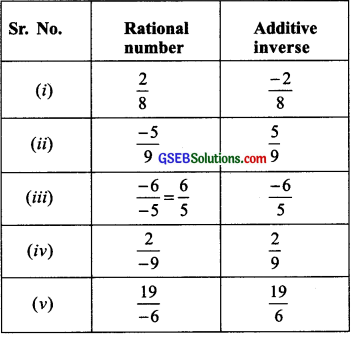Question 3.
Verify that – (-x) = x for:

1. x = $$\frac { 11 }{ 15 }$$
2. x = – $$\frac { 13 }{ 17 }$$

Solution: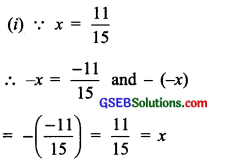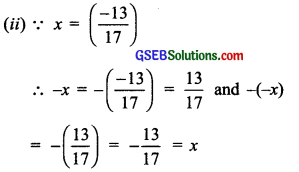Question 4.
Find the multiplicative inverse of the following:

1. – 13
2. $$\frac { -13 }{ 19 }$$
3. $$\frac { 1 }{ 5 }$$
4. $$\frac { -5 }{ 8 }$$ × $$\frac { -3 }{ 7 }$$
5. -1 × $$\frac { -2 }{ 5 }$$
6. -1

Solution: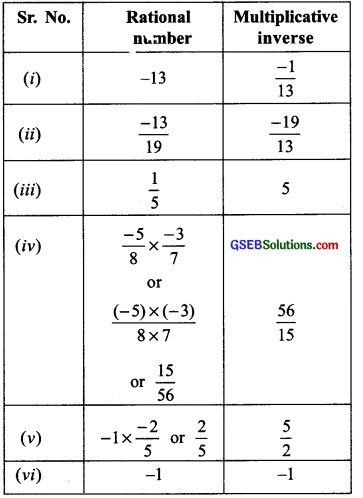Question 5.
Name the property under multiplication used in each of the following:

1. $$\frac { 1 }{ 2 }$$ × 1 = 1 × $$\frac { -4 }{ 5 }$$ = – $$\frac { 4 }{ 5 }$$
2. – $$\frac { 13 }{ 17 }$$ × $$\frac { 13 }{ 17 }$$
3. $$\frac{-19}{29} \times \frac{29}{-19}$$ = 1

Solution: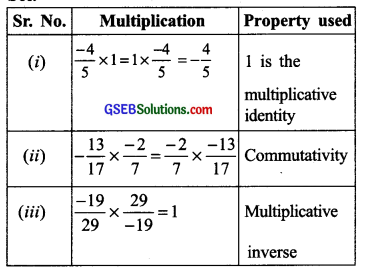Question 6.
Multiply $$\frac { 6 }{ 13 }$$ by the reciprocal of $$\frac { -7 }{ 16 }$$
Solution: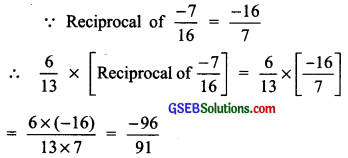Question 7.
Tell what property allows you to compute
$$\frac { 1 }{ 3 }$$ ×$$\left(6 \times \frac{4}{3}\right)$$ as $$\left(\frac{1}{3} \times 6\right) \times \frac{4}{3}$$
Solution:
In computing $$\frac { 1 }{ 3 }$$ ×$$\left(6 \times \frac{4}{3}\right)$$ as $$\left(\frac{1}{3} \times 6\right) \times \frac{4}{3}$$ , we use the associativity.Question 8.
Is $$\frac { 8 }{ 9 }$$ the multiplicative invers of -1$$\frac { 1 }{ 8 }$$ ?
Why or Why not?
Solution:
Since, -1$$\frac { 1 }{ 8 }$$ = $$\frac { -9 }{ 8 }$$ and $$\frac { 8 }{ 9 }$$ × $$\frac { 8 }{ 9 }$$ × $$\frac { -9 }{ 8 }$$ = -1
[Which is not equal to 1]
∴ $$\frac { 8 }{ 9 }$$ is not the multiplicative invers of $$\frac { -9 }{ 8 }$$
[∴ The product of $$\frac { -9 }{ 8 }$$ and its multiplication invers must be equal to 1]

Question 9.
Is 0.3 the multiplicative inverse of 3 $$\frac { 1 }{ 3 }$$ ? Why or why not?
Solution:
0.3 = $$\frac { 3 }{ 10 }$$ and 3$$\frac { 1 }{ 3 }$$ = $$\frac { 10 }{ 3 }$$
and, multiplicative invers of 3 $$\frac { 1 }{ 3 }$$ or $$\frac { 10 }{ 3 }$$ = $$\frac { 3 }{ 10 }$$ = 0.3
∴the multiplicative inverse of 3$$\frac { 1 }{ 3 }$$ is 0.3

Question 10.
Write:

1. The rational number that does not have a reciprocal.
2. The rational numbers that are equal to their reciprocals.
3. The rational number that is equal to its negative.

Solution:

1. The rational number zero (0) does not have a reciprocal.
2. The rational numbers 1 and (-1) are equal to their reciprocals respectively.
3. ∵ [A rational number] + [Negative of the rational number] = 0 [ ∵  =  = 0 ]
So, Negative of 0 is 0. Hence, 0 is equal to its negative.Question 11.
Fill in the blanks:

1. Zero has _______ reciprocal
2. The numbers _______ and _____ are their own reciprocals.
3. The reciprocal of -5 is ______
4. Reciprocal of $$\frac { 1 }{ x }$$ , where x ≠ 0 is ______.
5. The reciprocal of a positive rational number is always a _______
6. The reciprocal of a positive rational number is ______

Solution:

1. Zero has no reciprocal.
2. The numbers 1 and -1 are their own reciprocals.
3. The reciprocal of -5 is $$\frac { -1 }{ 5 }$$
4. The reciprocal of $$\frac { 1 }{ x }$$ where x ≠ 0 is x.
5. The product of two rational numbers is always a rational number.
6. The reciprocal of a positive rational number is positive.